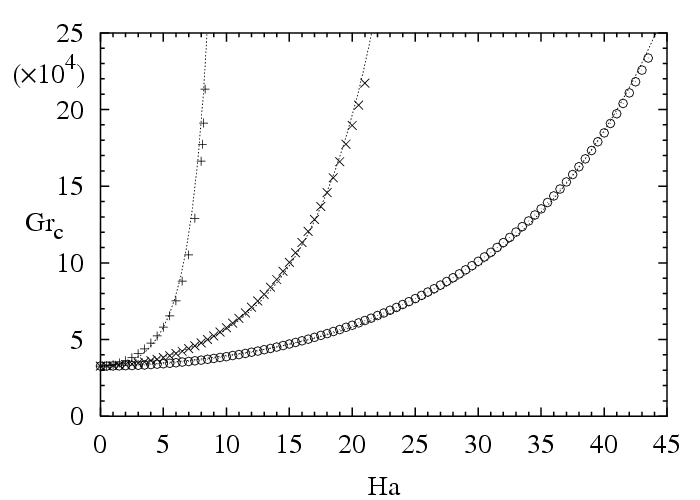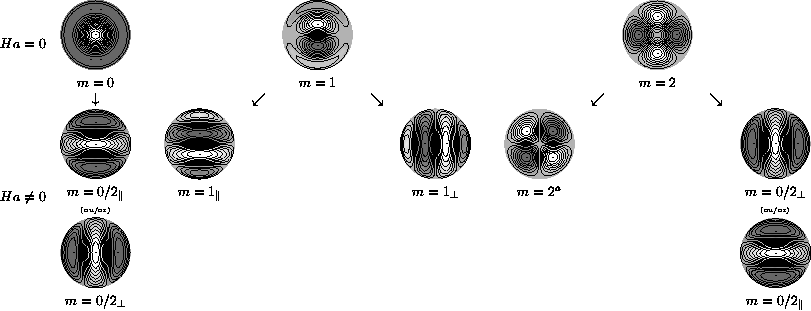# Fluid Mechanics and Acoustics Laboratory - UMR 5509

LMFA - UMR 5509
Laboratoire de Mécanique des Fluides et d’Acoustique
Lyon
France

## Convection and instabilities in presence of magnetic field

Hamda Ben Hadid, Daniel Henry, R. Touihri, S. Kaddeche, T. Mullin, A. Juel, T. Lyubimova

In the case of electrically conducting materials as semi-conductors, the control of convection during material processing can be obtained by applying a static or a rotating magnetic field.

The rotating magnetic field can be used to uniformize the solute concentration near the interface. Our studies in this field (collaborations with T. Lyubimova (Russia)) principally concern the action of the rotating magnetic field on the horizontal longitudinally heated cylindrical cavity, action on the convection and on the instabilities which are triggered [B15, A98].

The static magnetic field can be used to control the convection and the oscillatory instabilities in the melt in order to get better quality materials. Our studies in this field were numerous. The first studies concerned the characterization of the convection braking and flow modifications obtained by application of a magnetic field in horizontal parallelepipedic and cylindrical cavities which are longitudinally heated (collaboration with T. Mullin and A. Juel from university of Manchester (U.K.)) [A23, A29, A33, A34, A39, A50, A55].

We then developed studies to understand the stabilizing effect of the magnetic field on the flows. Different studies are concerned by situations where the applied temperature gradient is horizontal: stabilization by magnetic field of the flow instabilities in an infinite fluid layer (Hadley flow configuration studied by linear analysis in collaboration with S. Kaddeche from INSAT of Tunis (Tunisia)) [A34, A58, A59, A60, A63], in a transversally confined fluid layer (collaboration with T. Lyubimova from Perm (Russia)) [A90, A91], and in a parallelepipedic cavity (collaboration with Manchester) [A86, A87]. Another study is concerned by the stabilization by magnetic field of the Rayleigh-Bénard instabilities in cylindrical cavities and has been performed with a continuation method with R. Touihri (LAMSIN, Tunis) [A31, A40, A45, A47, A48, A52, A94, A100, A101].Variation of the oscillatory thresholds ($Gr_c$) as a function of the magnetic field intensity ($Ha$) for a laterally heated three-dimensional cavity (Aspect ratios: longitudinal $A_x=4$, transverse $A_y=2$; $Pr=0.026$). Three orientations of the magnetic field are considered: vertical (+) (very strong stabilization), transverse (X) (strong stabilization), longitudinal (circles) (slowest stabilization) [A87].Evolution of the three first Fourier modes (which are the more unstable in a cylindrical cavity heated from below) when a horizontal magnetic field is applied (horizontal direction on the figure). The figure gives the three modes $m=0$, $m=1$ and $m=2$ for $Ha=0$ (the modes $m=1$ et $m=2$ are defined to within a rotation), and the five unique possibilities for $Ha$ non zero, which correspond to orientated structures (the plots give the vertical velocity at mid-height in the cavity).Stabilisation of the first three critical modes which appear in a cylindrical cavity heated from below with a free surface when a horizontal magnetic field is applied ($Ra_c$ as a function of $Ha$). The modes with the roll axis parallel to the magnetic field are less stabilized (the plots give the vertical velocity at mid-height in the cavity).